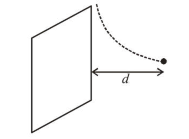# A particle of charge q and mass mQuestion:

A particle of charge $q$ and mass $m$ is moving with a velocity$v \hat{i}(v \neq 0)$ towards a large screen placed in the Y-Zplane at a distance $d$. If there is a magnetic field $\vec{B}=B_{0} \hat{k}$, the minimum value of $v$ for which the particle will not hit the screen is:

1. (1) $\frac{q d B_{0}}{3 m}$

2. (2) $\frac{2 q d B_{0}}{m}$

3. (3) $\frac{q d B_{0}}{m}$

4. (4) $\frac{q d B_{0}}{2 m}$

Correct Option: , 3

Solution:

(3) In uniform magnetic field particle moves in a circular path, if the radius of the circular path is ' $r$ ', particle will not hit the screen.$r=\frac{m v}{q B_{0}}$          $\left[\because \frac{m v^{2}}{r}=q v B_{0}\right]$

Hence, minimum value of $v$ for which the particle will not hit the screen.

$v=\frac{q B_{0} d}{m}$